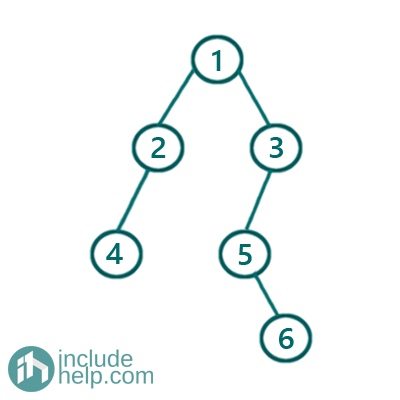# Print the path from Root to the given node

In this article, we are going to see how to find the path from the root to the given node, if found?
Submitted by Radib Kar, on August 20, 2020

In this article, we are going to see that given a node how to find the path from the root to that given node? If the given node doesn't exist then print 'Node doesn't exist". In the below example tree,If the given node is 6,

The path found will be 1->3->5->6

If the given node is 10,

Then the output will be "Node doesn't exist" as we can’t find the given node.

```Function rootToNode(TreeNode* node, TreeNode* nodeToFind)
//base cases
1)  If current node is NULL
Return Empty string;
2)  If the given node is NULL
Return Empty string;

Declare final_path="" which will store the root to node path if the node exists;
if node found in the tree
return the path stored in final_path
otherwise, return an empty string
```

To find the node along with storing the path, we use the function rootToNodeRecur(node, "", final_path, nodeToFind)

```Function rootToNodeRecur(TreeNode* node,string current_path, string& final_path, TreeNode* nodeToFind):
1)  if node is NULL
return false;
2)  if node is found store the path in final_path & return true
3)  intermediate path up to now is,  current_path+=string(node->val)+"->";
4)  If any of the subtrees contain the node return true
Otherwise
return false;
End Function
```

C++ Implementation:

```#include <bits/stdc++.h>
using namespace std;

// tree node is defined
class TreeNode {
public:
int val;
TreeNode* left;
TreeNode* right;
TreeNode(int data)
{
val = data;
left = NULL;
right = NULL;
}
};

bool rootToNodeRecur(TreeNode* node, string current_path, string& final_path, TreeNode* nodeToFind)
{
//if node is NULL
if (!node)
return false;

//if node is found store the path in final_path
if (node->val == nodeToFind->val) {
final_path = current_path + to_string(nodeToFind->val);
return true;
}

//intermediate path upto now
current_path += to_string(node->val) + "->";

//recur for the left subtree
if (rootToNodeRecur(node->left, current_path, final_path, nodeToFind))
return true;

//recur for the right subtree if node not found in the left subtree
if (rootToNodeRecur(node->right, current_path, final_path, nodeToFind))
return true;

return false;
}

string rootToNode(TreeNode* node, TreeNode* nodeToFind)
{
//base cases
//1.if current node is NULL
if (!node)
return "";

//2. If the given node is NULL
if (!nodeToFind)
return "";

//final_path will store the root to node path if node exists
string final_path = "";

//current_path stores intermediate paths
string current_path = "";

//if node found in the tree
//return the path stored in final_path
//otherwise return empty string
if (rootToNodeRecur(node, current_path, final_path, nodeToFind))
return final_path;
else
return "";
}

int main()
{
//building the tree like example 1
TreeNode* root = new TreeNode(1);

root->left = new TreeNode(2);
root->right = new TreeNode(3);
root->left->left = new TreeNode(4);
root->right->left = new TreeNode(5);
root->right->left->right = new TreeNode(6);

TreeNode* nodeToFind = new TreeNode(6);
string path = rootToNode(root, nodeToFind);
if (path != "")
cout << "Root to node path is: " << path << endl;
else

nodeToFind = new TreeNode(10);
path = rootToNode(root, nodeToFind);

if (path != "")
cout << "Root to node path is: " << path << endl;
else

return 0;
}
```

Output:

```Root to node path is: 1->3->5->6
```

Dry run with the examples:

```Input tree: Tree same as example
Node to Find: 6

In the main we call:
rootToNode(1,"",6)
so call to rootToNode(1,"",6)
The current node is not NULL
Current node value is not the same as the value of the node to find
The intermediate path up to now is "1->"

Recur for its subtrees to find the node

First, we check the left subtree and it will return false
since we are unable to find the node 6 there

So, recur for the right subtree and if you continue
the dry run you will find the path "1->3->5->6"

For the second node with value 10, both the subtrees will return false
and thus it will return an empty string from the function rootToNode()
```

Languages: » C » C++ » C++ STL » Java » Data Structure » C#.Net » Android » Kotlin » SQL
Web Technologies: » PHP » Python » JavaScript » CSS » Ajax » Node.js » Web programming/HTML
Solved programs: » C » C++ » DS » Java » C#
Aptitude que. & ans.: » C » C++ » Java » DBMS
Interview que. & ans.: » C » Embedded C » Java » SEO » HR
CS Subjects: » CS Basics » O.S. » Networks » DBMS » Embedded Systems » Cloud Computing
» Machine learning » CS Organizations » Linux » DOS
More: » Articles » Puzzles » News/Updates